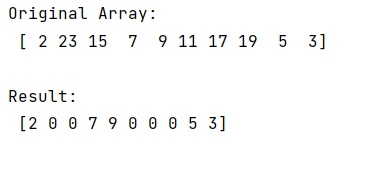# Set numpy array elements to zero if they are above a specific threshold

Learn, how to set numpy array elements to zero if they are above a specific threshold in Python?
Submitted by Pranit Sharma, on January 19, 2023

NumPy is an abbreviated form of Numerical Python. It is used for different types of scientific operations in python. Numpy is a vast library in python which is used for almost every kind of scientific or mathematical operation. It is itself an array which is a collection of various methods and functions for processing the arrays.

## NumPy Array - Setting elements to zero if they are above a specific threshold

Suppose that we are given a NumPy array that contains some integer values and we need to check if some value is greater than some threshold value, we will replace this value with 0.

For this purpose, we will follow the simple indexing technique. We will use the [ ] on this NumPy array and inside this, we will write our specific condition, if the condition is met, we will assign these values as 0.

Let us understand with the help of an example,

## Python code to set numpy array elements to zero if they are above a specific threshold

```# Import numpy
import numpy as np

# Creating a numpy array
arr = np.array([2, 23, 15, 7, 9, 11, 17, 19, 5, 3])

# Display original array
print("Original Array:\n",arr,"\n")

# Replacing those values which are
# greater than a value
arr[arr > 10] = 0

# Display result
print("Result:\n",arr)
```

Output:What's New (MCQs)

Top Interview Coding Problems/Challenges!

IncludeHelp's Blogs

Languages: » C » C++ » C++ STL » Java » Data Structure » C#.Net » Android » Kotlin » SQL
Web Technologies: » PHP » Python » JavaScript » CSS » Ajax » Node.js » Web programming/HTML
Solved programs: » C » C++ » DS » Java » C#
Aptitude que. & ans.: » C » C++ » Java » DBMS
Interview que. & ans.: » C » Embedded C » Java » SEO » HR
CS Subjects: » CS Basics » O.S. » Networks » DBMS » Embedded Systems » Cloud Computing
» Machine learning » CS Organizations » Linux » DOS
More: » Articles » Puzzles » News/Updates